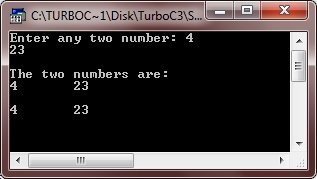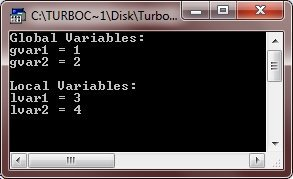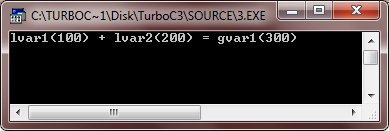# C++ Variable Scope

Variable scope represents the scope of a variable, that is, where they can be used in a C++ program. Basically there can be two scope of a variable in a C++ program. The first one is local variables and the second one is global variables. Let's take a look.

## C++ Local Variables

Local variables are those variables which are declared inside a function/block. Here is an example of local variables in C++, demonstrating the variable scope in C++:

```/* C++ Variable Scope - Local Variable */

#include<iostream.h>
#include<conio.h>
void main()
{
clrscr();
int a = 100, b = 200, c;
c = a + b;
cout<<"a(100) + b(200) = c("<<c<<")";
getch();
}```

Here is the sample output of the above C++ program:Let's take another program also demonstrating variable scope in C++:

```/* C++ Variable Scope - Local Variable */

#include<iostream.h>
#include<conio.h>
void main()
{
clrscr();
int num1, num2;
cout<<"Enter any two number: ";
cin>>num1>>num2;
cout<<"\nThe two numbers are:\n";
cout<<num1<<"\t"<<num2;
if(num1<100 || num2<100)
{
int localvar1, localvar2;   // local variables declared
localvar1=num1;
localvar2=num2;
cout<<"\n\n"<<localvar1<<"\t"<<localvar2;
}
getch();
}```

Here is the sample run of this C++ program:If you try to use localvar1 and localvar2, out of the if block (where they are declared locally) like:

```/* C++ Variable Scope - Wrong use of Local Variables */

#include<iostream.h>
#include<conio.h>
void main()
{
clrscr();
int num1, num2;
cout<<"Enter any two number: ";
cin>>num1>>num2;
cout<<"\nThe two numbers are:\n";
cout<<num1<<"\t"<<num2;
if(num1<100 || num2<100)
{
int localvar1, localvar2;   // local variables declared
localvar1=num1;
localvar2=num2;
}
cout<<"\n\n"<<localvar1<<"\t"<<localvar2;   // error occurred..!!
getch();
}```

Then, four error message will be displayed as shown in this figure:As you can see from the above C++ program, local variables are destroyed upon exit of the scope where they are declared. So they becomes unknown outside their block. Now let's discuss about global variables in C++

## Global Variables

Global variables are those variables which are defined outside of all the functions/block. Here is an example using global variables, helps you in understanding variable scope in C++:

```/* C++ Variable Scope - Global Variable */

#include<iostream.h>
#include<conio.h>

int gvar1, gvar2;

void main()
{
clrscr();
int lvar1, lvar2;

gvar1 = 1;
gvar2 = 2;
lvar1 = 3;
lvar2 = 4;

cout<<"Global Variables:\n";
cout<<"gvar1 = "<<gvar1<<"\n";
cout<<"gvar2 = "<<gvar2<<"\n";
cout<<"\nLocal Variables:\n";
cout<<"lvar1 = "<<lvar1<<"\n";
cout<<"lvar2 = "<<lvar2<<"\n";

getch();
}```

Here is the sample output of this C++ program:Here is another C++ program, also uses local and global variables both:

```/* C++ Variable Scope - Global Variable */

#include<iostream.h>
#include<conio.h>

int gvar1;

void main()
{
clrscr();
int lvar1, lvar2;
lvar1 = 100;
lvar2 = 200;
gvar1 = lvar1 + lvar2;
cout<<"lvar1(100) + lvar2(200) = gvar1("<<gvar1<<")";
getch();
}```

Here is the sample output of this C++ program:You can also declare local and global variable with same name. But remember, the local variable will take higher preference. More local variable, more preference. Here is an example:

```/* C++ Variable Scope - Global Variable */

#include<iostream.h>
#include<conio.h>

int var = 200;

void main()
{
clrscr();

/* local variable is going to declare
* with same name as global variable
* Local variables always having high
* priority than global variables.
* Therefore the value (100) initialized here
* will be printed, not 200.
*/

int var = 100;
cout<<var;
getch();
}```

Below is the sample output of the above C++ program:Tools
Calculator